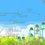# Some General Questions

1. The first term of a sequence is 2014. Each succeeding term is the sum of the cubes of the digits of the previous term. What is the 2014th term of the sequence?

2. In a triangle with integer side lengths, one side is three times as long as a second side, and the length of the third side is 17. What is the greatest possible perimeter of the triangle?

3. If real numbers a, b, c, d, e satisfy a + 1 = b + 2 = c + 3 = d + 4 = e + 5 = a + b + c + d + e + 3, what is the value of a^2 + b^2 + c^2 + d^2 + e^2

4. Let ABCD be a convex quadrilateral with perpendicular diagonals. If AB = 20, BC = 70 and CD = 90, then what is the value of DA?

5. A natural number k is such that k^2 < 2014 < (k + 1)^2. What is the largest prime factor of k?

6. Let S be a set of real numbers with mean M. If the means of the sets S∪{15} and S∪{15, 1} are M + 2 and M + 1, respectively, then how many elements does S have?

7. What is the smallest possible natural number n for which the equation x^2 − nx + 2014 = 0 has integer roots?

8. In a triangle ABC, X and Y are points on the segments AB and AC, respectively, such that AX : XB = 1 : 2 and AY : Y C = 2 : 1. If the area of triangle AXY is 10 then what is the area of triangle ABC?

9. Let XOY be a triangle with angleXOY = 90◦ . Let M and N be the midpoints of legs OX and OY , respectively. Suppose that XN = 19 and Y M = 22. What is XY ?Note by Vishruth Khare
6 years, 2 months ago

This discussion board is a place to discuss our Daily Challenges and the math and science related to those challenges. Explanations are more than just a solution — they should explain the steps and thinking strategies that you used to obtain the solution. Comments should further the discussion of math and science.

When posting on Brilliant:

• Use the emojis to react to an explanation, whether you're congratulating a job well done , or just really confused .
• Ask specific questions about the challenge or the steps in somebody's explanation. Well-posed questions can add a lot to the discussion, but posting "I don't understand!" doesn't help anyone.
• Try to contribute something new to the discussion, whether it is an extension, generalization or other idea related to the challenge.

MarkdownAppears as
*italics* or _italics_ italics
**bold** or __bold__ bold
- bulleted- list
• bulleted
• list
1. numbered2. list
1. numbered
2. list
Note: you must add a full line of space before and after lists for them to show up correctly
paragraph 1paragraph 2

paragraph 1

paragraph 2

[example link](https://brilliant.org)example link
> This is a quote
This is a quote
    # I indented these lines
# 4 spaces, and now they show
# up as a code block.

print "hello world"
# I indented these lines
# 4 spaces, and now they show
# up as a code block.

print "hello world"
MathAppears as
Remember to wrap math in $$ ... $$ or $ ... $ to ensure proper formatting.
2 \times 3 $2 \times 3$
2^{34} $2^{34}$
a_{i-1} $a_{i-1}$
\frac{2}{3} $\frac{2}{3}$
\sqrt{2} $\sqrt{2}$
\sum_{i=1}^3 $\sum_{i=1}^3$
\sin \theta $\sin \theta$
\boxed{123} $\boxed{123}$

Sort by:

All of these are from the Pre - RMO in Mumbai region, right?

- 6 years, 2 months ago

Yes !!

- 6 years, 2 months ago

If u have some more question of pre emo send to muramallaswaroop@gmail.com. ..... k?

- 6 years, 2 months ago

sure ... actually I too am hunting for these papers !! :)

- 6 years, 2 months ago

This Set has all of the Pre - RMO questions of 2014. You can also find them on the HBCSE's site I think.

- 6 years, 2 months ago

thanks ... If you have any more sets or papers of this kind then kindly send me the links or stuff... also HBCSE site copied ... thanks again ..!!!

- 6 years, 2 months ago

waiting fr ur email dude.......

- 6 years, 2 months ago

I said I have this pre rmo paper only ...you can check the websites suggested by Siddhartha Srivastava ..... kindly have a look. PS: I've got few more papers regarding class 10 and 11 course ..but i'll mail them to you after my exams are over .. so plz wait till 24th ..

- 6 years, 2 months ago

I wanted the papers so am asking since you have fr 10 th and 11 th pls do mail them earliest....pls

- 6 years, 2 months ago